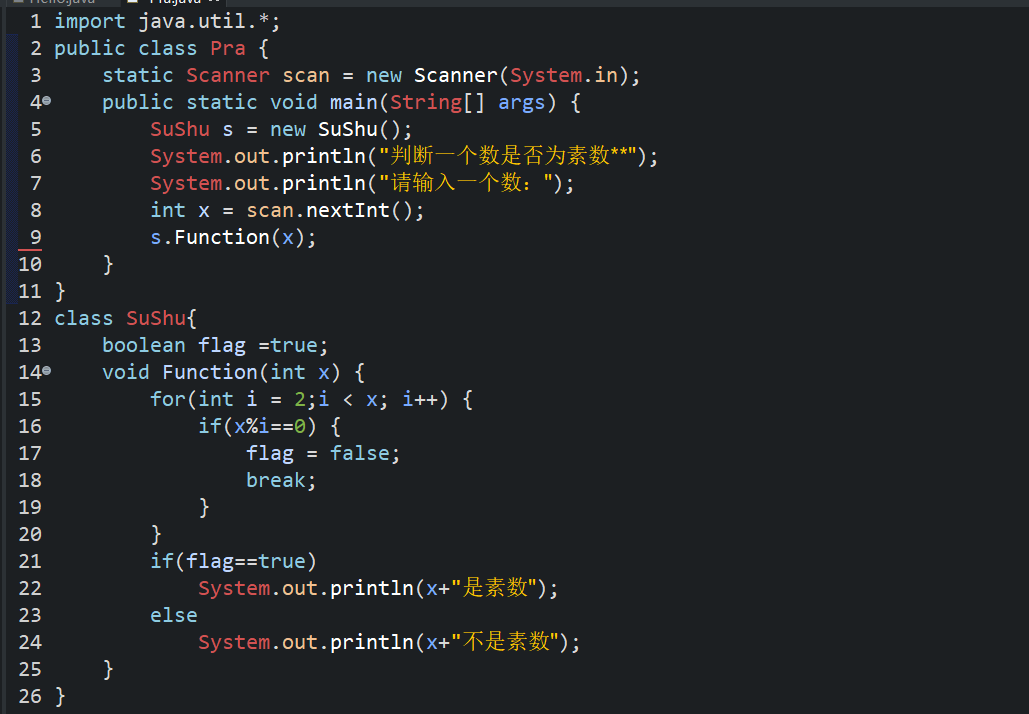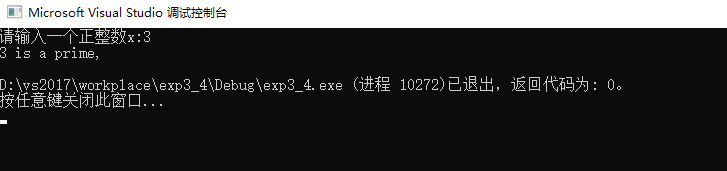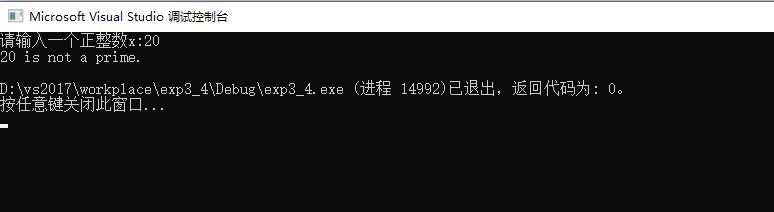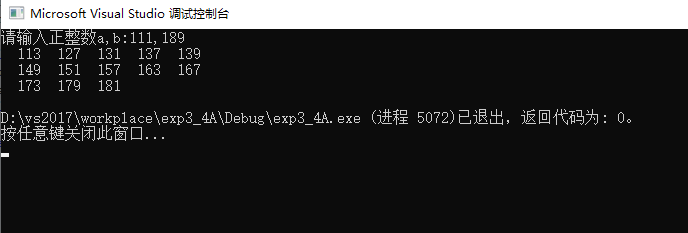• 判断一个数x是否为素数

千次阅读 2019-03-30 20:41:27
判断一个数x是否素数 思路： 若一个数是素数，则它的因数只有“1”和它本身。 采用循环，依次从2开始到这个数x-1取模，若期间有(x%i==0)，则这个数必然不是素数。 代码： import java.util.*; public class Pra {...

判断一个数x是否为素数

思路：
若一个数是素数，则它的因数只有“1”和它本身。
采用循环，依次从2开始到这个数x-1取模，若期间有(x%i==0)，则这个数必然不是素数。代码：

import java.util.*;
public class Pra {
static Scanner scan = new Scanner(System.in);
public static void main(String[] args) {
SuShu s = new SuShu();
System.out.println("判断一个数是否为素数**");
System.out.println("请输入一个数：");
int x = scan.nextInt();
s.Function(x);
}
}
class SuShu{
boolean flag =true;
void Function(int x) {
for(int i = 2;i < x; i++) {
if(x%i==0) {
flag = false;
break;
}
}
if(flag==true)
System.out.println(x+"是素数");
else
System.out.println(x+"不是素数");
}
}

*~~此文纯属记录作者学习日记，若有误导，纯属意外~ *

展开全文判断素数
• 用C语言编写自定义函数prime(int x)，判断x是否素数？int prime(int x){int i，kk=(int)sqrt( (double)x )for(i=2i&lt=ki )if(x%i==0)break// 如果完成所有循环，那么x为素数if(i&gtk)retrun 1elsereturn ...

用C语言编写自定义函数prime(int x)，判断x是否为素数？

int prime(int x)

{

int i，k

k=(int)sqrt( (double)x )

for(i=2i&lt=ki )

if(x%i==0)

break

// 如果完成所有循环，那么x为素数

if(i&gtk)

retrun 1

else

return 0

}

C语言，编写函数判断一个整数是否为素数，在主函数中调用该函数并输出100以内的全部素数？

参考代码：

#include &ltstdio.h&gt

int is_prime_number(int n)

{

int i

int result = 1

if(n==1)

return 0

for(i=2i&ltni )

{

if(n%i==0)

{

result = 0

break

}

}

return result

}

int main()

{

int n

printf("100以内的素数包括：")

for(n=1n&lt=100n )

{

if(is_prime_number(n))

{

printf("%d "，n)

}

}

printf("")

return 0

}

编写程序，其中自定义函数，用来判断一个整数是否为素数，主函数输入一个数，输出是否为素数？

自己动手写吧，这是最基本的能力啦.....(课本一般会有的)

C 编程，编写函数判断变量是否为素数？

多的我就不说了，函数如下(你只需把下面的代码贴在intmain()之前就行了，然后在开头加一个头文件：#include

boolisPrime(intx){

if(x==1)return0

for(inti=2i

if(x%i==0)return0

return1}

函数就是上面的，使用方法：比如你想判断n(这里n为正整数)是否为素数，只需运行isPrime(n)，如果n是素数，则刚才的函数返回1，否则返回0.

不懂在发站内信问我

编程用自定义函数求100到200之间的素数？

#include "stdio.h" int isPrime(int n)//判断n是否是素数，是返回1，不是返回0 { int i for(i=2i&lt=n/2i ) if(n%i==0) return 0 return 1 } int main() { int i printf("100到200之间的素数: ") for(i=100i&lt=200i ) if(isPrime(i)==1) printf("%d"，i) printf(" ") }

python编写一个函数.判断一个数是不是素数，然后调用该函数输出100以内的素数？

def is_prime(n): list_num = [] for i in range(2， n): for num in range(2， int(sqrt(n)) 1): if i % num == 0 and i != num: break elif i % num != 0 and num == (int(sqrt(n))): list_num.append(i) return list_num

展开全文• #include<iostream>#include<cstdio>#include<cmath>using namespace std;int a;int main(){ int p,b=0,flag=1; scanf("%d",&p);...i++) { if(p%i==0) { printf("...

#include<iostream>
#include<cstdio>
#include<cmath>
using namespace std;
int a;
int main()
{
int p,b=0,flag=1;
scanf("%d",&p);
for(int i=2;i<=p/2;i++)
{
if(p%i==0)
{
printf("composite");
return 0;
}
}
printf("prime");
return 0;
}

转载于:https://www.cnblogs.com/kuaileyongheng/p/6607152.html

展开全文• 判断正整数是否质数的三种方法 本文参考《如何判断一个正整数是否质数的三种方法 | 附Python程序》结合自身理解，作为笔记发布。如果对你有帮助，点赞关注哦！ 一、基本概念 质数（又称素数）： 一个大于1的...方法
• 判断从键盘读入的正整数x是否质数，并输出判断结果。 #include<stdio.h> #include<math.h> int main() { int x, k, i; printf("请输入一个正整数x:"); scanf_s("%d", &x); if (x <= 0) ...

判断从键盘读入的正整数x是否为质数，并输出判断结果

#include<stdio.h>
#include<math.h>
int main()
{
int x, k, i;
printf("请输入一个正整数x:");
scanf_s("%d", &x);
if (x <= 0)
printf("请输入一个正整数:");
else if (x == 1)
printf("%d is not a prime.\n", x);
else
{
//判断从2到平方根的数字是否都能被整除即可
k = (int)sqrt(x);
for (i = 2; i <= k; i++)
if (x%i == 0)
break;
if (i > k)
printf("%d is a prime,\n", x);
else
printf("%d is not a prime.\n", x);
}
return 0;
}

运行结果：在此基础上改写，找出规定范围（a,b）内的所有质数，并按每行5个的格式输出。（这里a，b变量从键盘输入并且范围为：10≤a≤b≤1000）

#include<stdio.h>
#include<math.h>
int main()
{
int x, k, i, count = 0, a, b;
printf("请输入正整数a,b:");
scanf_s("%d,%d", &a, &b);
if (a < 10 || b>1000 || a > b)
printf("请输入a和b使得10<=a<=b<=1000!");
else
for (x = a + 1; x < b; x++)
{
//判断是否是质数
k = (int)sqrt(x);
for (i = 2; i <= k; i++)
if (x%i == 0)
break;
//按照每行5个的格式输出
if (i > k)
{
count++;
printf("%5d", x);
if (count % 5 == 0)
printf("\n");
}
}
printf("\n");
return 0;
}

运行结果：展开全文c语言
• 给定一个正整数n ( >= 3), 判断是不是素数。 思路介绍 使用蒙特卡罗法算法结合费尔马小定理结合二次探测定理。 费尔马小定理：如果p是素数，则有 ap−1  mod  p=1a^{p-1} \; mod \; p = 1ap−1modp=1, a∈[2,p−...
• 本文实例讲述了Python编程判断一个正整数是否素数的方法。分享给大家供大家参考，具体如下： import string import math #判断是否素数的函数 def isPrime(n): if(n<2 xss=removed>2): for d in range(2,int...
• * 从2 到x-1 测试是否可以整除 循环 * 要循环 x-1 遍 * 当x很大时，循环次数约等于 x遍 */ static void checkPrime01(int x){ boolean prime = true; if(x == 1){ prime = false; } for (int i=2;i<x;i+...
• #define _CRT_SECURE_NO_WARNINGS #include<stdio.h> int is_prime(int x) { int j = 0; { for (j = 2; j < x; j++) if (x % j == 0) return 0;... printf("以下为100之内的素数:\n"); ...c++
• import java.util.Scanner;public class Test { // 输入一个整数判断是否质数 public static void main(String[] args) { System.out.println("请输入一个正整数"); Scanner input = new Scanner(System.in)...
• int fun(){int i,j,t=1,sum=0;for(i=100;i<=200;i++){for(j=2;jif(t){printf("%d\t",i);sum+=1;}t=1;}return sum;}main(){intnum;num=fun();printf("sum=%d",num);...}扩展资料编写函数fun()，函数的功能是：根据...
• 展开全部#include#includeint zshu(int x)//判断x是不是素数。32313133353236313431303231363533e59b9ee7ad9431333431353963zshu代表素数，即质数。{int i;i=2;if(x==2)//x是素数 。return 1;else if(x==1)//x不是...
• 判断素数输入一个正整数m，判断是否素数素数就是只能被1和自身整除的正整数，1不是素数，2是素数
• c语言判断是否素数程序代码

千次阅读 2021-05-19 13:05:31
#include bool isPrimeNum(int x){if (x == 1)return false;else if (x <= 0)return false;else if (x == 2)return true;else{for (int i = 2; i < x; i++){if (x%i == 0)return false;}return true;}}int mai...
• 在计算机程序中，算法是...下面小编向大家演示在python如何判断数字是否质数质数：一个大于1的自然数，除了1和它本身外，不能被其他自然数(质数)整除(2, 3, 5, 7等)，换句话说就是该数除了1和它本身以外不再有...
• 1）直观判断法最直观的方法，根据定义，因为质数除了1和本身之外没有其他约数，所以判断n是否质数，根据定义直接判断从2到n-1是否存在n的约数即可。C++代码如下：bool isPrime_1( int num ) { int t...质数 判断 算法
• 质数是与合数相对立的两个概念，二者构成了数论当中最基础的定义之一。基于质数定义的基础之上而建立的问题有很多世界级的难题，如哥德巴赫猜想等。算术基本定理证明每个大于1的正整数都可以写成素数的乘积，并且...
• 适合初学者的代码学习，有助于帮助初学者了解C语言，帮助其更快入门。
• 2)是否素数答案:3信息版本：手机版解决时间 2019-10-06 13:12已解决2019-10-05 20:25素数：除了1和它自身，没有其他因子最佳答案2019-10-05 21:10int main(){int a, i;scanf("%d", &a);for (i= 2; i < a; i...
• def is_prime(x):  if x in range(0,2):   return False  else:   if x>=2:   for n in range(2,x):   if x%n==0:   return False
• ruby判断一个数是否质数质数又称素数。一个大于1的自然数，如果除了1和它自身外，不能被其他自然数整除的数；（除0以外）否则称为合数 。根据算术基本定理，每一个比1大的整数，要么本身是一个质数，要么可以写成...
• *任意输入一个正整数判断是否质数 *质数：只能被1和本身整除 最小质数为2 */ #include &lt;stdio.h&gt; int main(void) { unsigned int num; printf("任意输入一个正整数:"); scanf(&...
• PHP 判断一个数是否是质数

千次阅读 2018-05-14 17:52:20
的整数可以表示为偶数(2x)和奇数(2x+1)，其中第二个if条件把偶数(2x)和能被3整除的数(3x)已过滤，则还剩下奇数中不被3整数的数(3x+1,3x+2)还没有判断。  2) 3x+1中的奇数为 2(3x+1)-1=6x+1和2(3x+1)+1=6x+3=3(2x+1)...PHP PHP判断是否是质数 素数
• python输入并判断一个数是否素数x=int(input("x\n")); i=2; for i in range(2,x+1): if(x%i==0): break;if(i==x and i。用python 判断一个数是否素数小编觉得小编的程序是对的但为什么没办法运行，那个弹出来的...
• param number: 测试该数字是否是超级素数幂 return: 如果不是就返回 False，如果是就返回 p 和 q 值 例如，输入125，返回（5，3） 代码： import math def get_prime(number): ''' 寻找小于number的所有的质数...
• 注意：1不是质数素数）； 质数的定义是： 只能够被1和本身整除的数 #include <stdio.h> int is_prime(int n){ if(n==1 ||n==0) return 1; for (int i = 2; i<n; i++) { if (n%i==0) { return 1; } ...c语言 算法
• 输出素数的个数。 样例输入： 3 2 3 4 样例输出： 2 这是我写的代码，输入小数据的时候没有问题，数一大就不行了，（因为math.h库里的函数sqrt精度不够，所以又写了一个求平方根函数）。 我听...c语言
• 输入一个正整数，判断是否素数 x=int(input("请输入一个正整数：")) if x<2: print("这个数不是素数！") else: for i in range(2,x): if x%i==0: print("该数不是素数！") break else: print("该数是...python 素数筛...